Alyssa Alyssa Quek 2018-04-28T10:36:06.847Z alyssaq.github.io/ Alyssa Quek Hexo Simple Movie Recommender Using SVD alyssaq.github.io/2015/20150426-simple-movie-recommender-using-svd/ 2015-04-26T19:13:00.000Z 2018-04-28T10:36:06.847Z Given a movie title, we’ll use Singular Value Decomposition (SVD) to recommend other movies based on user ratings.

Filtering and recommending based on information given by other users is known as collaborative filtering. The assumption is that people with similar movie tastes are most likely to give similar movie ratings. So, if I’m looking for a new movie and I’ve watched The Matrix, this method will recommend movies that have a similar rating pattern to The Matrix across a set of users.

## SVD Concept

The essence of SVD is that it decomposes a matrix of any shape into a product of 3 matrices with nice mathematical properties: $A = U S V^T$.

By lucid analogy, a number can decompose into 3 numbers to always have the smallest prime in the middle. E.g $24 = 3 \times 2 \times 4$ or $57 = 1 \times 3 \times 19$.

For the interested, I previously wrote a post on SVD visualisation to view the properties of the decomposition.

The result of the decomposition leaves us with an ordered matrix of singular values which encompass the variance associated with every direction. We assume that larger variances means less redundancy and less correlation and encode more structure about the data. This allows us to use a representative subset of user rating directions or principal components to recommend movies.

I highly recommend reading John Shlen’s tutorial on PCA and SVD (2014) to fully understand the mathematical properties of the two related methods.

## Simple Recommender

Python libraries we’ll be using:

import numpy as npimport pandas as pd

We’ll be using 2 files from the MovieLens 1M dataset: ratings.dat and movies.dat.

1) Read the files with pandas

data = pd.io.parsers.read_csv('data/ratings.dat',     names=['user_id', 'movie_id', 'rating', 'time'],    engine='python', delimiter='::')movie_data = pd.io.parsers.read_csv('data/movies.dat',    names=['movie_id', 'title', 'genre'],    engine='python', delimiter='::')

2) Create the ratings matrix of shape ($m \times u$) with rows as movies and columns as users

ratings_mat = np.ndarray(    shape=(np.max(data.movie_id.values), np.max(data.user_id.values)),    dtype=np.uint8)ratings_mat[data.movie_id.values-1, data.user_id.values-1] = data.rating.values

3) Normalise matrix (subtract mean off)

normalised_mat = ratings_mat - np.asarray([(np.mean(ratings_mat, 1))]).T

4) Compute SVD

A = normalised_mat.T / np.sqrt(ratings_mat.shape - 1)U, S, V = np.linalg.svd(A)

5) Calculate cosine similarity, sort by most similar and return the top N.

def top_cosine_similarity(data, movie_id, top_n=10):    index = movie_id - 1 # Movie id starts from 1    movie_row = data[index, :]    magnitude = np.sqrt(np.einsum('ij, ij -> i', data, data))    similarity = np.dot(movie_row, data.T) / (magnitude[index] * magnitude)    sort_indexes = np.argsort(-similarity)    return sort_indexes[:top_n]# Helper function to print top N similar moviesdef print_similar_movies(movie_data, movie_id, top_indexes):    print('Recommendations for {0}: \n'.format(    movie_data[movie_data.movie_id == movie_id].title.values))    for id in top_indexes + 1:        print(movie_data[movie_data.movie_id == id].title.values)

6) Select $k$ principal components to represent the movies, a movie_id to find recommendations and print the top_n results.

k = 50movie_id = 1 # Grab an id from movies.dattop_n = 10sliced = V.T[:, :k] # representative dataindexes = top_cosine_similarity(sliced, movie_id, top_n)print_similar_movies(movie_data, movie_id, indexes)
Recommendations for Toy Story (1995): Toy Story (1995)Toy Story 2 (1999)Babe (1995)Bug's Life, A (1998)Pleasantville (1998)Babe: Pig in the City (1998)Aladdin (1992)Stuart Little (1999)Secret Garden, The (1993)Tarzan (1999)

We can change k and use different number of principal components to represent our dataset. This is essentially performing dimensionality reduction.

## SVD and PCA relationship

Instead of computing SVD in step 4 above, the same results can be obtained by computing PCA using the eigenvectors of the co-variance matrix:

normalised_mat = ratings_mat - np.matrix(np.mean(ratings_mat, 1)).Tcov_mat = np.cov(normalised_mat)evals, evecs = np.linalg.eig(cov_mat)

We re-use the same cosine similarity calculation in step 5. Instead of the matrix V from SVD, we can use the eigenvectors computed from the co-variance matrix:

k = 50movie_id = 1 # Grab an id from movies.dattop_n = 10sliced = evecs[:, :k] # representative datatop_indexes = top_cosine_similarity(sliced, movie_id, top_n)print_similar_movies(movie_data, movie_id, top_indexes)
Recommendations for Toy Story (1995): Toy Story (1995)Toy Story 2 (1999)Babe (1995)Bug's Life, A (1998)Pleasantville (1998)Babe: Pig in the City (1998)Aladdin (1992)Stuart Little (1999)Secret Garden, The (1993)Tarzan (1999)

Exactly the same results!
In step 4 above, our input matrix $A$ has shape $u \times m$. The computation of V from SVD is the result of the eigenvectors of $A^T A$. The columns of V are the eigenvectors that correspond to the sorted eigenvalues in the diagonal of $S$.

By construction, $A^T A$ equals the covariance matrix of normalised_mat. Thus, the columns of $V$ are the principal components of normalised_mat. (Refer to section VI of John Shlen’s tutorial (2014) for the full mathematical proof of this relationship).

### Why use SVD over the covariance matrix?

• Its faster (Facebook published a fast randomized SVD)
• Singular values from SVD are sorted (we have to sort the eigenvalues in ascending order)
]]>
<p>Given a movie title, we’ll use <a href="http://mathworld.wolfram.com/SingularValueDecomposition.html" target="_blank" rel="noopener">Sing
Site Deployment With Gzipped Assets to AWS S3 Using CircleCI alyssaq.github.io/2015/deploy_gzip_site_s3/ 2015-04-16T23:02:00.000Z 2018-04-28T10:36:06.847Z I’ve been using Heroku and Google App Engine to host most of my web applications. This time, I decided to use Amazon S3 to serve a static website.

## Amazon S3 setup

After creating your S3 bucket, click on the Properties button.

1) Enable static website hosting. For single page apps, specify the same error and index document.2) Under Permissions > Edit CORS Configuration, make all files in this bucket accessible to the public by pasting this XML:

<?xml version="1.0" encoding="UTF-8"?><CORSConfiguration xmlns="http://s3.amazonaws.com/doc/2006-03-01/">    <CORSRule>        <AllowedOrigin>*</AllowedOrigin>        <AllowedMethod>GET</AllowedMethod>        <MaxAgeSeconds>3000</MaxAgeSeconds>        <AllowedHeader>Authorization</AllowedHeader>        <AllowedHeader>Content-Type</AllowedHeader>    </CORSRule></CORSConfiguration>

There are 2 save buttons. Per section and global save, so click em all!

## Gzip assets with gulp

Unfortunately, S3 does not automatically compress assets. Here is their verbose instructions for serving compressed files.

Basically,

1. gzip assets
2. Set header with Content-Encoding: gzip

Yes, my JS and CSS assets are always gzipped and ancient browsers (such as IE < 5, Firefox < 0.9.5) are not supported. Code for the future!

I gzip as part of my gulp build.

Heres the relevant gulp build task:

gulp.task('build', ['clean'], function () {  gulp.start('default', function() {    return gulp.src('dist/**/*')      .pipe($.size({title: 'build', gzip: true})) .pipe($.if('*.js', $.gzip({ append: false }))) .pipe($.if('*.css', $.gzip({ append: false }))) .pipe(gulp.dest('dist')) })}) I run clean (to delete destination folder), then the default task (to browserify, babelify, concatenate, minify, replace, etc) and then gzip only the minified JS and CSS assets. $.gzip({ append: false }) replaces the file with the gzipped version and pipes to the dest folder. Setting to true appends .gz.

The npm gulp packages involved in this step:

$npm install gulp-load-plugins gulp-if gulp-gzip  ## Auto-deploy with CircleCI In the CircleCI project settings > AWS Permissions, set your Amazon Access Key ID and Secret Access Key from an IAM user with S3 PutObject and GetObject permissions (Amazon S3 permission list). I started off with a user that had permissions to all S3 actions and then pruned later. To deploy the app to S3, circleCI runs gulp build and sync the dist folder with AWS CLI sync. My circle.yml: machine: node: version: 0.12.0dependencies: post: - npm install -g gulp-cli && gulp builddeployment: production: branch: master commands: - sudo pip install awscli - aws s3 sync dist/ s3://newcleus-app --exclude "*" --include "*.css" --include "*.js" --content-encoding gzip --cache-control public,max-age=30672000 - aws s3 sync dist/ s3://newcleus-app --exclude "*.js" --exclude "*.css" Under deployment, the first aws s3 command adds the Content-Encoding: gzip and Cache-Control headers to the JS and CSS assets. The second command syncs the remaining files. ## Overall On my Mac, I use Cyberduck to view all files in all my S3 buckets. In the end, while not as friendly as Heroku, I was pretty happy with this auto-deployment to S3. ]]> <p>I’ve been using <a href="https://www.heroku.com" target="_blank" rel="noopener">Heroku</a> and <a href="https://cloud.google.com/appengin Face Morpher Talk at FOSS Asia alyssaq.github.io/2015/face-morpher-talk-at-foss-asia/ 2015-03-15T00:08:00.000Z 2018-04-28T10:36:06.846Z I just presented at FOSS Asia (free open source software) conference. I talked about face morphing and averaging using Python. ]]> <p>I just presented at FOSS Asia (free open source software) conference.<br>I talked about face morphing and averaging using Python.</p> <p> Singular Value Decomposition (SVD) Visualisation alyssaq.github.io/2015/singular-value-decomposition-visualisation/ 2015-02-24T09:03:00.000Z 2018-04-28T10:36:06.846Z “The SVD is absolutely a high point of linear algebra” (Gilbert Strang, Kai Borre) This mathematical technique has been used across various industries and example applications include recommender systems (Netflix prize), face and object recognition, risk modelling in equity options and identifying genes in brain imaging that make up Parkinson disease. ## Definition SVD is a matrix factorisation and decomposes a matrix of any size into a product of 3 matrices: $$A = U S V^T$$$A$:$n \times m$: number of records as rows and number of dimensions/features as columns.$U$:$n \times n$: orthogonal matrix containing eigenvectors of$AA^T$.$S$:$n \times m$: ordered singular values in the diagonal. Square root of eigenvalues associated with$AA^T$or$A^TA$(its the same).$V$:$m \times m$: orthogonal matrix containing eigenvectors of$A^TA$. Orthogonal matrix: square matrix where columns make$90^\circ$angles between each other and its inner dot product is zero.$Q^TQ = QQ^T = I$and$Q^T=Q^{-1}$. Orthonormal matrix: orthogonal matrix where columns are unit vectors. ## Example matrix For this post, Im going to use the same matrices from my post on eigenvectors and eigenvalues. We have a matrix$x = \begin{bmatrix}
-10 & -10 & 20 & 20\\
-10 & 20 & 20 & -10
\end{bmatrix}$and a transformation matrix$A = \begin{bmatrix}
1 & 0.3 \\
0.45 & 1.2
\end{bmatrix}$. In the plot below, the dashed square shows$x$as the corners and the transformed matrix$Ax$as the solid shape.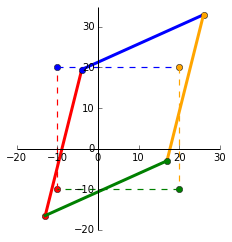Python’s numpy provides us with a handy svd function: In:A = np.matrix([[1, 0.3], [0.45, 1.2]])U, s, V = np.linalg.svd(A)Out:U:[[-0.58189652 -0.81326284] [-0.81326284 0.58189652]]s:[ 1.49065822 0.71444949]V:[[-0.63586997 -0.77179621] [-0.77179621 0.63586997]] Lets verify some properties of the SVD matrices. # Verify calculation of A=USVIn: np.allclose(A, U * np.diag(s) * V)Out: True# Verify orthonormal properties of U and V. (Peformed on U but the same applies for V).# 1) Dot product between columns = 0In: np.round([np.dot(U[:, i-1].A1, U[:, i].A1) for i in xrange(1, len(U))])Out: [ 0. 0.]# 2) Columns are unit vectors (length = 1)In: np.round(np.sum((U*U), 0))Out: [[ 1. 1. 1.]]# 3) Multiplying by its transpose = identity matrixIn: np.allclose(U.T * U, np.identity(len(U)))Out: True ## Geometric interpretation of SVD Transformation of a matrix by$U S V^T$can be visualised as a rotation and reflection, scaling, rotation and reflection. We’ll see this as a step-by-step visualisation. #### (1)$V^Tx$We can see that multiplying by$V^T$rotates and reflects the input matrix$x$. Notice the swap of colours red-blue and green-yellow indicating a reflection along the x-axis.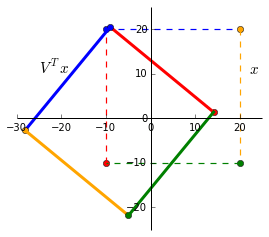#### (2)$SV^Tx$Since$S$only contains values on the diagonal, it simply scales the matrix. The singular values$S$are ordered in descending order so$s_1 > s_2 > … > s_n$.$V$rotates the matrix to a position where the singular values now represent the scaling factor along the x and y-axis. This is now the V-basis. The black dots shows this ($V^Tx$is dashed and$SV^Tx$solid): •$V^Tx$intercepts the x-axis at$x = -25.91$. • Largest singular value of$s_1 = 1.49$is applied and$s_1x = -38.61$. •$V^Tx$intercepts the y-axis at$y = 12.96$. • Smallest singular value of$s_2 = 0.71$is applied and$s_2y = 9.20$.While not shown, there is a similar rotation and scaling effect in the U-basis with$Ux$and$SUx$. #### (3)$USV^Tx$Finally,$U$rotates and reflects the matrix back to the standard basis. As expected, this is exactly the same as$Ax$.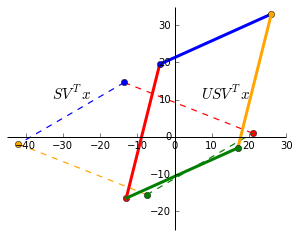## Eigen decomposition vs singular value decomposition In an eigen-decomposition,$A$can be represented by a product of its eigenvectors$Q$and diagonalised eigenvalues$\Lambda$: $$A = Q \Lambda Q^{-1}$$. • Unlike an eigen-decomposition where the matrix must be square ($n \times n$), SVD can decompose a matrix of any dimension. • Column vectors in$Q$are not always orthogonal so the change in basis is not a simple rotation.$U$and$V$are orthogonal and always represent rotations (and reflections). • Singular values in$S$are all real and non-negative. The eigenvalues in$\Lambda$can be complex and have imaginary numbers. ## Resources SVD ties together the core concepts of linear algebra — matrix transformations, projections, subspaces, change of basis, eigens, symmetric matrices, orthogonalisation and factorisation. For a complete proof and backgound, I highly recommend the entire MIT Linear Algebra lectures by Gilbert Strang. I also found the examples and proofs for SVD and QR factorisation from the University of Texas’ MOOC useful. Other posts covering SVD visualisations and its applications: ]]> <p><a href="https://books.google.com.sg/books?id=MjNwWUY8jx4C&amp;pg=PA259" target="_blank" rel="noopener">“The SVD is absolutely a high poi Computing the Axes or Orientation of a Blob alyssaq.github.io/2015/computing-the-axes-or-orientation-of-a-blob/ 2015-01-14T09:30:00.000Z 2019-11-14T17:34:06.616Z How do we find the major and minor axes of a blob? We look at using 2 popular methods to obtain the same result: 1. Covariance matrix and eigenvectors, eigenvalues (partial PCA). 2. Raw image moments ## 1. Covariance matrix and eigens An eigenvalue tells us the scaling magnitude along the direction of its corresponding eigenvector. Check my post on understanding eigens for the visual intuition. Covariance matrix is a square and symmetric matrix that summarises the variance between two variables. So, with a set of$(x, y)$points, the covariance matrix is 2x2:$ C = \begin{bmatrix}
variance(x,x) & variance(x,y) \\
variance(x,y) & variance(y,y)
\end{bmatrix}$, where the variance of$(x, y)$and$(y, x)are the same. Principal component analysis (PCA) is a method commonly used for dimensionality reduction. The eigenvectors of the covariance matrix are called principal components. Heres a thorough tutorial on PCA and applied to computer vision (Lindsay Smith, 2002). Eigenvectors with the largest eigenvalue of a covariance matrix gives us the direction along which the data has the largest variance. ### Computation Outline 1. Matrix of points. We’ll be dealing with 2D points so our matrix is 2xm. The 1st row are the x-coordinates, 2nd row are the y-coordinates and m indicates the number of points. 2. Subtract the mean for each point. Calculate the mean of the row of x-coordinates. For each x-point, subtract the mean from it. Do the same for the y-coordinates. Mean subtraction minimises the mean square error of approximating the data and centers the data. 3. Covariance matrix calculation. Calculate the 2x2 covariance matrix. 4. Eigenvectors, eigenvalues of covariance matrix. Find the 2 eigen-pairs of our dataset. 5. Rearrange the eigen-pairs. Sort by decreasing eigenvalues. 6. Plot the principal components Steps 1-5 are the beginning crux to performing dimensionality reduction with PCA. ### Code Time We’ll use a binary image as input and our matrix of points are the indices to the white pixels. Heres the image:First, the libraries we’ll be using: import numpy as npimport matplotlib.pyplot as pltimport scipy.miscimport skimage.filter 1) Read the image in grey-scale. We want the indexes of the white pixels to find the axes of the blob. img = misc.imread('oval.png', flatten=1)y, x = np.nonzero(img) 2) Subtract mean from each dimension. We now have our 2xm matrix. x = x - np.mean(x)y = y - np.mean(y)coords = np.vstack([x, y]) 3 & 4) Covariance matrix and its eigenvectors and eigenvalues cov = np.cov(coords)evals, evecs = np.linalg.eig(cov) 5) Sort eigenvalues in decreasing order (we only have 2 values) sort_indices = np.argsort(evals)[::-1]x_v1, y_v1 = evecs[:, sort_indices] # Eigenvector with largest eigenvaluex_v2, y_v2 = evecs[:, sort_indices] 6) Plot the principal components. The larger eigenvector is plotted in red and drawn twice as long as the smaller eigenvector in blue. scale = 20plt.plot([x_v1*-scale*2, x_v1*scale*2], [y_v1*-scale*2, y_v1*scale*2], color='red')plt.plot([x_v2*-scale, x_v2*scale], [y_v2*-scale, y_v2*scale], color='blue')plt.plot(x, y, 'k.')plt.axis('equal')plt.gca().invert_yaxis() # Match the image system with origin at top leftplt.show()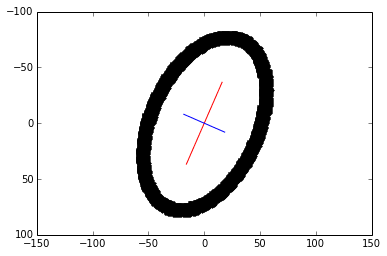7) Bonus! We vertically-align the blob based on the major axis via a linear transformation. An anti-clockwise rotating transformation matrix has the general form:\begin{bmatrix}
cos \theta & -sin \theta \\
sin \theta & cos \theta
2 & 0 \\
0 & 3
\end{bmatrix}$. In : A = np.matrix([[2, 0], [0, 3]])In : evals, evecs = np.linalg.eig(A)Out: evals: [ 2. 3.] evecs: [[ 1. 0.] [ 0. 1.]] The first eigenvalue, 2, is associated with the eigenvector in the first column (1, 0). Equation of the line through$(0,0)$and$(1,0)$is$y = 0$. So, any point on this line when multiplied by the transformation matrix$A$, will be scaled by 2. Similarly, for the second eigenvalue, any point on the line$x = 0$will be scaled by 3. ## Visualising eigenvalues and eigenvectors Lets use a more involved transformation matrix$A = \begin{bmatrix}
1 & 0.3 \\
0.45 & 1.2
\end{bmatrix}$. For visualisation, we’ll plot a matrix$x = \begin{bmatrix}
-10 & -10 & 20 & 20\\
-10 & 20 & 20 & -10
\end{bmatrix}$and its transformed state after it has been multiplied with$A$.The dashed square shows the original matrix$x$and the transformed matrix$Ax$. Now we’ll see where the eigens come into play. In : A = np.matrix([[1, 0.3], [0.45, 1.2]])In : evals, evecs = np.linalg.eig(A)Out: evals: [ 0.71921134 1.48078866] evecs: [[-0.73009717 -0.52937334] [ 0.68334334 -0.84838898]] To plot the eigenvectors, we calculate the gradient: In : x_v1, y_v1 = evecs[:,0].getA1() x_v2, y_v2 = evecs[:,1].getA1()In : m1 = y_v1/x_v1 # Gradient of 1st eigenvector m2 = y_v2/x_v2 # Gradient of 2nd eigenvectorOut : m1 = -0.936, m2 = 1.603 # Round to 3dp So, our eigenvectors, which span all vectors along the line through the origin, have the equations:$y = -0.936x$($e1$) and$y = 1.603x$($e2$). This is plotted in blue and red below.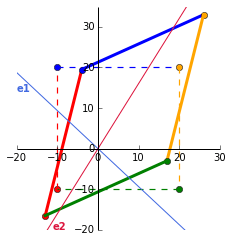What does this eigen plot tell us? The point where the first eigenvector line$e1$intercepts the original matrix is$p1 = (10.68, -10)$. Multiplying this point by the corresponding eigenvalue of 0.719 OR by the transformation matrix$A$, yields$T(p1) = (7.684, -7.192). \begin{equation} \begin{aligned} A \times p1 \ &= \begin{bmatrix} 1 & 0.3 \\ 0.45 & 1.2 \end{bmatrix} & \times \begin{bmatrix} 10.68 \\ -10 \end{bmatrix} &= \begin{bmatrix} 7.684 \\ -7.192 \end{bmatrix} \\ \lambda \times p1 \ &= 0.719 & \times \begin{bmatrix} 10.68 \\ -10 \end{bmatrix} &= \begin{bmatrix} 7.684 \\ -7.192 \end{bmatrix} \\ \end{aligned} \end{equation} \\ \therefore Ax = \lambda x Doing this fore2$will show the same calculation. As this eigenvector is associated with the largest eigenvalue of 1.481, this is the maximum possible stretch when acted by the transformation matrix. To complete the visuals, we’ll plot$p1$(the intercept with$e1$),$p2$(the intercept with$e2$) and their transformed point$T(p1)$and$T(p2)$. Gist for the full plot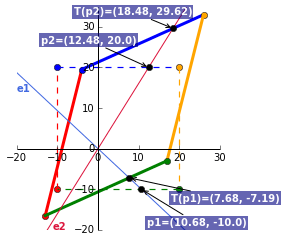## Eigendecomposition We can rearrange$Ax = \lambda x$to represent$A$as a product of its eigenvectors and eigenvalues by diagonalising the eigenvalues: $$A = Q \Lambda Q^{-1}$$ with$Q$as the eigenvectors and$\Lambda$as the diagonalised eigenvalues. Using the values from the example above: $$\begin{bmatrix} 1 & 0.3 \\ 0.45 & 1.2 \end{bmatrix} = \begin{bmatrix} -0.730 & -0.529 \\ 0.683 & -0.848 \end{bmatrix} \begin{bmatrix} 0.719 & 0 \\ 0 & 1.481 \end{bmatrix} \begin{bmatrix} -0.730 & -0.529 \\ 0.683 & -0.848 \end{bmatrix}^{-1}$$ To check this in Python: In : A = np.matrix([[1, 0.3], [0.45, 1.2]])In : evals, evecs = np.linalg.eig(A)In : np.allclose(A, evecs * np.diag(evals) * np.linalg.inv(evecs))Out: True Next up, we’ll connect the eigen decomposition to another super useful technique, the singular value decomposition. ]]> <p>Eigenvectors and eigenvalues are used in many engineering problems and have applications in <a href="http://www.cipa.dcu.ie/papers/spie_9 Visualising Matrices and Affine Transformations With Python alyssaq.github.io/2015/visualising-matrices-and-affine-transformations-with-python/ 2015-01-02T08:00:00.000Z 2018-04-28T10:36:06.844Z Here, we visualise matrix linear and affine transformations to set the foundation in understanding more awesome computer vision techniques (still a learning process for me). There is an assumed knowledge of the core mathematical concepts in matrices and vectors. If a refresher is needed, I highly recommend Khan Academy’s Precalculus course. I also recommend Khan Academy’s Linear Algebra course for further learning. ## Linear vs Affine transform Quick distinction. Linear transformations are fixed around the origin (scaling, rotating, skewing). Affine transformations are a linear function followed by a translation. ## Visualising a matrix Points in 2D space can be written as (3, 13), (5, 15). A set of 2D vectors can be written in a matrix as$\begin{bmatrix}
3 & 5 \\
13 & 15
\end{bmatrix}$with each column representing a data point. So, matrix$P$=$\begin{bmatrix}
0 & 0 & 20 & 20\\
0 & 20 & 20 & 0
\end{bmatrix}$consists of 4 points. Lets plot it in Python. First, the libraries: import numpy as npimport matplotlib.pyplot as plt I’m going to draw a line between the points but you should picture the line as a row of many points. Heres the function to plot the matrix that will be reused throughout this post: def plot_points(matrix, ls='--', lw=1.2, colors=None): x_points, y_points = matrix size = len(x_points) colors = ['red', 'blue', 'orange', 'green'] if not None else colors for i in range(size): plt.plot(x_points[i], y_points[i], color=colors[i], marker='o') plt.plot([x_points[i], x_points[(i+1) % size]], [y_points[i], y_points[(i+1) % size]], color=colors[i], linestyle=ls, linewidth=lw) Now, we plot our matrix,$P$. x_points = np.array([0, 0, 20, 20])y_points = np.array([0, 20, 20, 0])matrix = np.array([x_points, y_points])colors = ['red', 'blue', 'orange', 'green']size = len(x_points)plot_points(matrix, colors)plt.ylim([-5,25])plt.xlim([-5,25])plt.axes().set_aspect('equal')plt.show() And our matrix$P$=$\begin{bmatrix}
0 & 0 & 20 & 20\\
0 & 20 & 20 & 0
\begin{bmatrix} 3 \\ 13 \end{bmatrix} = \begin{bmatrix} 3 \\ 13 \end{bmatrix}$. Each column in the indentity matrix are called basis vectors (linearly independent and equations solve for 0). These will be super useful in deriving and visualising the transformation matrices. ## Scaling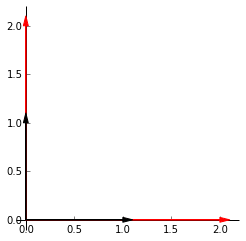Lets say we want to scale our points by 2. The plot on the left shows the identity matrix in black and our end goal in red. Heres the github gist for the plot. The first vector along the _x_-axis,$\begin{bmatrix} 1 \\ 0 \end{bmatrix}$has to be multipled by 2 to get to$\begin{bmatrix} 2 \\ 0 \end{bmatrix}$. The same is applied to the second vector along the _y_-axis. Our end matrix is$\begin{bmatrix} 2 & 0 \\ 0 & 2 \end{bmatrix}$. To generalise, we can multiply a matrix by$\begin{bmatrix} k1 & 0 \\ 0 & k2 \end{bmatrix}$to achieve scaling in 2D space.$k1$and$k2$can be the same or different to obtain different scalings in the different dimensions.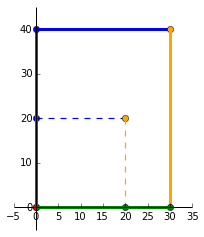Lets try scaling our matrix$P$by multiplying it with the matrix:$\begin{bmatrix}
1.5 & 0 \\
0 & 2
\end{bmatrix}$As expected, we can see that the original square (dashed lines) has been scaled by 1.5 in the positive _x_ direction and scaled by 2 in the positive _y_ direction. The resultant matrix is:$\begin{bmatrix}
1.5 & 0 \\
0 & 2
\end{bmatrix} \times
\begin{bmatrix}
0 & 0 & 20 & 20\\
0 & 20 & 20 & 0
\end{bmatrix} =
\begin{bmatrix}
0 & 0 & 30 & 30\\
0 & 40 & 40 & 0
-1 & 0 \\
0 & 1
\end{bmatrix}$and it’ll mirror along the _y_-axis. And the visuals!## Skewing Lets skew the identity matrix by +50%. The vector along the _x_-axis stays the same while the vector along the _y_-axis shifts _k_ = 0.5 to the right. Our resultant matrix is now$\begin{bmatrix}
1 & 0.5 \\
0 & 1
\end{bmatrix}$and a skewing transformation matrix can be generalised as$\begin{bmatrix}
1 & k \\
0 & 1
\end{bmatrix}$. So, doing$\begin{bmatrix}
1 & 0.5 \\
0 & 1
\end{bmatrix} \times
\begin{bmatrix}
0 & 0 & 20 & 20\\
0 & 20 & 20 & 0
\end{bmatrix}$will skew$P$by 50%.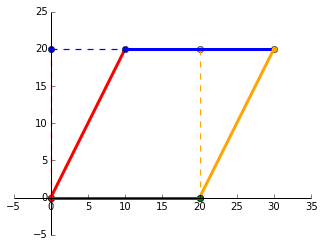## RotatingMy favourite linear transformation. Using the trusty SOH CAH TOA acronym, we can see that (1, 0) on the _x_-axis lands on$(cos \theta, sin \theta)$and (0, 1) on the _y_-axis lands on$(-sin \theta, cos \theta)$when rotated by positive$\theta$. Thus, our rotating transformation matrix has the general form:$\begin{bmatrix}
cos \theta & -sin \theta \\
sin \theta & cos \theta
\end{bmatrix}$. Lets plot$P$when it is rotated by$-30^\circ$. Note the change in negative$sin$for clockwise rotation. Our matrices involved in this transformation are:$\begin{bmatrix}
0.866 & 0.5 \\
-0.5 & 0.866
\end{bmatrix} \times
\begin{bmatrix}
0 & 0 & 20 & 20\\
0 & 20 & 20 & 0
\end{bmatrix} =
\begin{bmatrix}
0 & 10 & 27.32 & 17.32\\
0 & 17.32 & 7.32 & -10
\end{bmatrix}$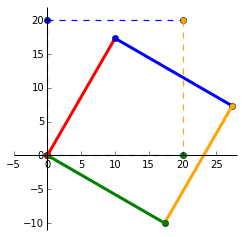## Translating Lastly, the affine transform to translate a matrix. To do this, we need to represent a 2-vector$(x, y)$to a 3-vector$(x, y, 1)$. A translation matrix has the form$\begin{bmatrix}
1 & 0 & t1 \\
0 & 1 & t2 \\
0 & 0 & 1
\end{bmatrix}$where$t1$and$t2$translates a vector in the _x_ and _y_ direction. Lets translate$P$to the left by 75% and downwards by 25%. Unlike the linear transformations where the scalars are multipliers, the affine scalars specify the distance to be translated.$\begin{bmatrix}
1 & 0 & -15 \\
0 & 1 & -5 \\
0 & 0 & 1
\end{bmatrix} \times
\begin{bmatrix}
0 & 0 & 20 & 20\\
0 & 20 & 20 & 0 \\
1 & 1 & 1 & 1
\end{bmatrix}$A = np.matrix([[1, 0, -0.75*20], [0, 1, -0.25*20], [0, 0, 1]]) P = np.matrix([[0, 0, 20, 20], [0, 20, 20, 0], [1, 1, 1, 1]]) translated_matrix = A*P  translated_matrix: matrix([[ -0.75, -0.75, 19.25, 19.25], [ -0.25, 19.75, 19.75, -0.25], [ 1. , 1. , 1. , 1. ]])## Transformation matrix Heres a summary of the transformation matrices (courtesy of wikipedia). Notice that a 2x2 linear transformation matrix becomes a 3x3 transformation matrix by padding it with 0s and a 1 at the bottom-right corner. So, for vectors in 3D ($\mathbb{R}^3$) space, its linear transformation matrix is 3x3 and its affine transformation matrix (usually called without the affine) is 4x4 and so on for higher dimensions.]]> <p>Here, we visualise matrix linear and affine transformations to set the foundation in understanding more awesome computer vision technique 2015 alyssaq.github.io/2015/2015/ 2015-01-01T08:24:00.000Z 2018-04-28T10:36:06.844Z 2014 Review Travelled to 10 different countries in Europe with Seb as the rider and myself as the pillion on his motorbike. Fell in love with the Alps. Did 4 trips from Singapore to Malaysia to hunt waterfalls. The 4th one spanned over 3 days to Cameron Highlands. Skied twice - first at Niseko, Japan; then at Thredbo, Australia. Joined a group of astronomy enthusiasts in Mersing and spent 2 nights engaging in some spectacular stargazing and astrophotography. Learnt node.js, python’s scipy stack, front-end development, devops, databases, APIs. Read one novel. Freelanced for the year and earned a third of my usual salary from the previous year. Spent 2 months obsessed over a super nutritious liquid blend, bentoblend. I still drink this for breakfast a couple of times a week. I had various depressing and lonely moments and various liberating and eye-opening moments. My perspective on life changed the most this year and I’ll continue learning and working to my fullest. ## Don’t just do, understand Learning college/university mathematics was hard. I remember being bogged down by the mathematical symbols and definitions. I had memorised the process of solving equations without understanding how it worked. I do the same with machine learning - applying neural networks and gradient boosted trees without understanding how it transforms my data. As Richard Feynman puts it: “There is a difference between knowing the name of something and knowing something”. This was also featured in the chapter O Americano, Outra Vez of his book Surely You’re Joking, Mr. Feynman!: Adventures of a Curious Character (I highly recommend reading it!). My aim for this year is to read more on my new a kindle paperwhite (which I named R2-D2) and understand more about the world! I want to do more scientific Python, computer vision, GPU programming, web apps, blog more and get out more often. ]]> <h2 id="2014-Review"><a href="#2014-Review" class="headerlink" title="2014 Review"></a>2014 Review</h2><p>Travelled to 10 different countrie Understanding Hough Transform With Python alyssaq.github.io/2014/understanding-hough-transform/ 2014-12-15T00:18:00.000Z 2018-04-28T10:36:06.843Z The Hough transform (Duda and Hart, 1972), which started out as a technique to detect lines in an image, has been generalised and extended to detect curves in 2D and 3D. Here, we understand how an image is transformed into the hough space for line detection and implement it in Python. ## How it works - gradient-intercept parameter space A line in Cartesian form has the equation: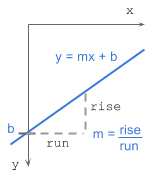y = mx + bwhere: m = gradient or slope of the line (rise/run) b = y-intercept Given a set of edge points or a binary image indicating edges, we want to find as many lines that connect these points in image space. Say we have 2 edge points ($x_1, y_1$) and ($x_2, y_2$). For each edge point at various gradient values ($m$=-0.5, 1.0, 1.5, etc), we calculate the corresponding$b$values. The image below shows the various lines through an edge point in image space and the plot of these lines in parameter space. Points which are collinear in the cartesian image space will intersect at a point in$m$-$b$parameter space.All points on a line in image space intersect at a common point in parameter space. This common point ($m$,$b$) represents the line in image space. Unfortunately, the slope,$m$, is undefined when the line is vertical (division by 0!). To overcome this, we use another parameter space, the hough space. ## How it works - angle-distance parameter space A line in Polar coordinate system has the equation: ρ = x cos θ + y sin θwhere:ρ (rho) = distance from origin to the line. [-max_dist to max_dist]. max_dist is the diagonal length of the image. θ = angle from origin to the line. [-90° to 90°] If you’re interested in how$\rho$is derived, I’ve laid out the maths at the bottom extras section: deriving rho. To explain the hough transform, I’ll use a simple example. I’ve created an input binary image of size 30 x 30 pixels with points at (5, 25) and (20, 10) shown below. The image is transformed to the hough space by calculating$\rho$with a point at each angle from$-90^\circ$to$90^\circ$(negative angles are anti-clockwise starting horizontally from the origin and positive angles are clockwise). The points in hough space make a sinusoidal curve.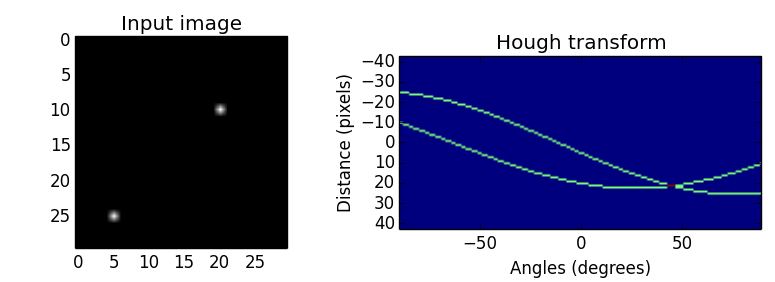The value of$\rho$at various angles for the 2 edge points: point$-90^\circ$$-45^\circ$$0^\circ$$45^\circ$$90^\circ$(5, 25)-25-1452125 (20, 10)-107202110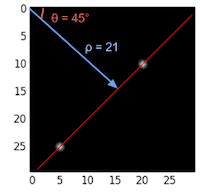We see that the curves in hough space intersect at$45^\circ$with$\rho=21$. Curves generated by collinear points in the image space intersect in peaks$(\rho, \theta)$in the Hough transform space. The more curves intersect at a point, the more “votes” a line in image space will receive. We’ll see this in the next implementation section. ## Algorithm steps 1. Corner or edge detection. (E.g. using canny, sobel, adaptive thresholding). The resultant binary/grey image will have 0s indicating non-edges and 1s or above indicating edges. This is our input image. 2. Rho range and Theta range creation.$\rho$ranges from -max_dist to max_dist where max_dist is the diagonal length of the input image.$\theta$ranges from$-90^\circ$to$90^\circ$. You can have more or less bins in the ranges to tradeoff accuracy, space and speed. E.g. Every third angle in$-90^\circ$to$90^\circ$to reduce from 180 to 60 values. 3. Hough accumulator of$\theta$vs$\rho$. It is a 2D array with the number of rows equal to the number of$\rho$values and the number of columns equal to the number of$\theta$values. 4. Voting in the accumulator. For each edge point and for each$\theta$value, find the nearest$\rho$value and increment that index in the accumulator. Each element tells how many points/pixels contributed “votes” for potential line candidates with parameters$(\rho, \theta)$. 5. Peak finding. Local maxima in the accumulator indicates the parameters of the most prominent lines in the input image. Peaks can be found most easily by applying a threshold or a relative threshold (values equal to or greater than some fixed percentage of the global maximum value). ## Code Time Full code on github import numpy as npdef hough_line(img): # Rho and Theta ranges thetas = np.deg2rad(np.arange(-90.0, 90.0)) width, height = img.shape diag_len = np.ceil(np.sqrt(width * width + height * height)) # max_dist rhos = np.linspace(-diag_len, diag_len, diag_len * 2.0) # Cache some resuable values cos_t = np.cos(thetas) sin_t = np.sin(thetas) num_thetas = len(thetas) # Hough accumulator array of theta vs rho accumulator = np.zeros((2 * diag_len, num_thetas), dtype=np.uint64) y_idxs, x_idxs = np.nonzero(img) # (row, col) indexes to edges # Vote in the hough accumulator for i in range(len(x_idxs)): x = x_idxs[i] y = y_idxs[i] for t_idx in range(num_thetas): # Calculate rho. diag_len is added for a positive index rho = round(x * cos_t[t_idx] + y * sin_t[t_idx]) + diag_len accumulator[rho, t_idx] += 1 return accumulator, thetas, rhos Usage: # Create binary image and call hough_lineimage = np.zeros((50,50))image[10:40, 10:40] = np.eye(30)accumulator, thetas, rhos = hough_line(image)# Easiest peak finding based on max votesidx = np.argmax(accumulator)rho = rhos[idx / accumulator.shape]theta = thetas[idx % accumulator.shape]print "rho={0:.2f}, theta={1:.0f}".format(rho, np.rad2deg(theta)) rho=0.50, theta=-45 Hough transform (and the faster probabilistic version) is available in openCV and scikit-image. ## Extensions Hough transform can be extended to detect circles of the equation$r^2 = (x − a)^2 + (y − b)^2$in the parameter space,$\rho = (a, b, r)$. Furthermore, it can be generalized to detect arbitrary shapes (D. H. Ballard, 1981). Another approach is the Progressive Probabilistic Hough Transform (Galamhos et al, 1999). The algorithm uses random subsets of voting points in the accumulator and checks for the longest segment of pixels with minimum gaps. Line segments that exceed a minimum length threshold are added to the list. This returns the beginning and end point of each line segment in the image. It has 3 thresholds: a minimum number of votes in the Hough accumulator, a maximum line gap for merging and a minimum line length. ## Extras ### Deriving rho: ρ = x cos θ + y sin θ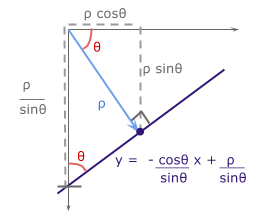With basic trigonometry, we know that for right-angled triangles,$sin \theta = {opposite\over hypotenuse}$and$cos \theta = {adjacent \over hypotenuse}$. We want to convert the cartesian form$y = mx + b$with parameters$(m, b)$to polar form with parameters$(\rho, \theta)$. The line from the origin with distance$\rho$has a gradient of${sin \theta \over cos \theta}$. The line of interest, which is perpendicular to it, will have a negative reciprocal gradient value of${-cos \theta \over sin \theta}$. For$b$, the y-intercept of the line of interest,$sin \theta = {\rho \over b}$. With$m = {-cos \theta \over sin \theta}$and$b = {\rho \over sin \theta}$, we get$\rho = x \ cos \theta + y \ sin \theta$. ]]> <p>The Hough transform <a href="http://www.ai.sri.com/pubs/files/tn036-duda71.pdf" target="_blank" rel="noopener">(Duda and Hart, 1972)</a>, Installing OpenCV on Mac OSX With Homebrew alyssaq.github.io/2014/installing-opencv-on-mac-osx-with-homebrew/ 2014-08-18T21:24:00.000Z 2018-04-28T10:36:06.843Z • Install the package manager Homebrew. It beats MacPorts any day. • Go ahead and install OpenCV: $ brew install opencv
• If all is well, it should be available at:

/usr/local/Cellar/opencv/2.4.9
• Create a symlink to the compiled OpenCV files. If you don’t mind setting up your PYTHONPATH and messing up python2 and python3 support, you may use brew link opencv. Otherwise, I recommend doing the manual symlink to your Python 2.7 site-packages.

$sudo ln -s /usr/local/Cellar/opencv/2.4.9/lib/python2.7/site-packages/cv.py /Library/Python/2.7/site-packages/cv.py$ sudo ln -s /usr/local/Cellar/opencv/2.4.9/lib/python2.7/site-packages/cv2.so /Library/Python/2.7/site-packages/cv2.so 
• Check that it works!

$ipythonPython 2.7.9[GCC 4.2.1 (Apple Inc. build 5666) (dot 3)] on darwinType "help", "copyright", "credits" or "license" ...In : import cv2In : img = cv2.imread('path-to-image/helloworld.jpg')In : cv2.imshow('Test image', img) • To check locations of site-packages folders: In : import site; site.getsitepackages()Out:['/Library/Frameworks/Python.framework/Versions/2.7/lib/python2.7/site-packages', '/Library/Frameworks/Python.framework/Versions/2.7/lib/site-python', '/Library/Python/2.7/site-packages'] ]]> <ol> <li>Install the package manager <a href="http://brew.sh/" target="_blank" rel="noopener">Homebrew</a>. It beats MacPorts any day.</li> How to Run SlimerJS and PhantomJS With CircleCI alyssaq.github.io/2014/how-to-run-slimerjs-and-phantomjs-with-circleci/ 2014-05-25T22:56:00.000Z 2018-04-28T10:36:06.842Z Goal Automatically run user-driven tests (BDD) with SlimerJS for testing on Gecko (firefox) and PhantomJS for WebKit (chrome/safari) on the continuous integration platform, CircleCI. ### Auto testing on CircleCI CircleCI’s test environment is preinstalled with CasperJS and PhantomJS but does not have SlimerJS installed. To get started, all you need is a circle.yml file in your repository, activate it in your CircleCI account and it’ll automatically run npm test if you’re using NodeJS. I also use it for my Python applications. Running unit tests and just PhantomJS tests is simple and documented on their site but getting SlimerJS working took a little more Googling and trial-and-error. Heres how I set it up. ### Custom commands to use SlimerJS on CircleCI Firstly, in the circle.yml file, specify custom commands to download the SlimerJS standalone installer (currently at 0.9.1). Extract the files and move them to a known directory. Moving the files to a known directly is the key! test: pre: - curl -k -L -o slimerjs.tar.bz2 http://download.slimerjs.org/v0.9/0.9.1/slimerjs-0.9.1-linux-x86_64.tar.bz2 - tar -jxf slimerjs.tar.bz2 - mv slimerjs-0.9.1$HOME/slimerjs

I do this in the test:pre section to have SlimerJS available before running the tests.

Next, add the required environment variables to CircleCI.

machine:  environment:    SLIMERJSLAUNCHER: $(which firefox) PATH:$PATH:$HOME/slimerjs Just ensure that the PATH environment contains the same directory as where you extracted the SlimerJS files. Heres my full circle.yml file with auto-deployment to Heroku and a web hook to my circleCI 2 slack herokuapp with names removed. machine: environment: SLIMERJSLAUNCHER:$(which firefox)        PATH: $PATH:$HOME/slimerjstest:  pre:    - bower install    - curl -k -L -o slimerjs.tar.bz2 http://download.slimerjs.org/v0.9/0.9.1/slimerjs-0.9.1-linux-x86_64.tar.bz2    - tar -jxf slimerjs.tar.bz2    - mv slimerjs-0.9.1 $HOME/slimerjsdeployment: production: branch: master heroku: appname: my-production-herokuapp staging: branch: develop heroku: appname: my-staging-herokuappnotify: webhooks: - url: https://my-circleci2slack.herokuapp.com/ If all is good, you should see green lights in all the rows in circleCI:]]> <h2 id="Goal"><a href="#Goal" class="headerlink" title="Goal"></a>Goal</h2><p>Automatically run user-driven tests (BDD) with <a href="http:/ Window Tiling in OS X alyssaq.github.io/2014/window-titling-in-os-x/ 2014-05-13T23:16:00.000Z 2018-04-28T10:36:06.842Z Discovered window tiling for my Mac and absolutely love it! Its open-source, light-weight and does what I need: spectacleapp Shortcuts to snap any application to left-half, right-half, quarter, thirds. Its awesome! ]]> <p>Discovered window tiling for my Mac and absolutely love it!</p> <p>Its open-source, light-weight and does what I need:<br><a href="http:/ How Do I Return a HTTP Response to Caller and Continue Processing alyssaq.github.io/2014/how-do-I-return-a-http-response-to-caller-and-continue-processing/ 2014-04-02T18:33:00.000Z 2018-04-28T10:36:06.842Z Scenario I have an analysis service that is invoked by posting data via its API. Since the processing could be long-running, it is best to return a response to the caller and continue with the data. ## Goal Return a 200 HTTP response to the caller upon receiving valid data. After returning the response, continue processing the data and post the results to another endpoint. ## Potential solutions ## Python multithreading Between multithreading and multiprocessing, using threads was sufficient for my requirements. I ended up using Python’s threading library. Below is the code gist to handle POST requests and continue processing with threads. Tested with python 2.7 and python 3.3 #!/usr/bin/env python# -*- coding: utf-8 -*-""" Sample multithreading with bottle.pyRequirements: requests, bottleTo run:$ python app.pyTo post data, open another command shell and type:$curl -X POST -H "Content-Type: application/json" -d '{"data":"test"}' http://127.0.0.1:8080/process -D-"""import bottleimport jsonimport requestsfrom threading import ThreadPOSTBACK_URL = 'http://127.0.0.1:8080/postprocessed'@bottle.post('/postprocessed')def postprocessed_handle(): print('Received data at postprocessed') return bottle.HTTPResponse(status=200, body="Complete postprocessed")def process_data(data): # do processing... result = json.dumps(data) print('Finished processing') requests.post(POSTBACK_URL, data=result, headers={'Content-Type':'application/json'})@bottle.post('/process')def process_handle(): data = bottle.request.json.get('data', False) print('Received data at process: ' + data) if not data: return bottle.HTTPResponse(status=400, body="Missing data") #Spawn thread to process the data t = Thread(target=process_data, args=(data, )) t.start() #Immediately return a 200 response to the caller return bottle.HTTPResponse(status=200, body="Complete process")if __name__ == '__main__': bottle.run() ## Terminologies ### multithreading vs multiprocessing • A thread is the smallest sequence of instructions within a process • A process is an instance of a computer program that is being executed. ThreadsProcesses Share address spaceSeparate address space Share process state, memory and other resources Carries more state information thread lifetime, context switching and synchronisation costs are lower slower context switching between processes than threads Exist as subsets of a processTypically independent. Insulated from each other by the OS An error in a thread can kill all the other threads in the same process An error in a process may not affect another process ]]> <h2 id="Scenario"><a href="#Scenario" class="headerlink" title="Scenario"></a>Scenario</h2><p>I have an analysis service that is invoked by Ready Player One - Summary and After-Effects alyssaq.github.io/2014/ready-player-one--summary-and-aftereffects/ 2014-03-10T22:46:00.000Z 2018-04-28T10:36:06.841Z You have to read Ready Player One (2011) by Ernest Cline! I have a major soft spot for utopian and dystopian future societies and characters. Throw in immersive virtual reality, artificial intelligence, pervasive computing vision, haptic technologies, Japanese culture and I’m in virtual heaven. Progress in technology is exponential and we’ll be able to merge with our technology within the next decade. No, no alien invasion. ## Welcome to Ready Player One In the dystopian 2044, a free-to-use virtual universe known as OASIS has become a daily refuge from the ugly real world and school systems are available online. When the creator, Halliday, dies without heirs, he leaves his$240 billion fortune and OASIS control to the finder of his Easter Egg.
To win, players have to unravel a series of riddles and video games which have been built around Halliday’s obsession of 80’s pop culture. We follow Wade Watt’s convolutions of his race towards the Egg in this highly entertaining, thrilling and addictive game within a novel.

As someone who often takes refuge from the real world by spending hours coding, reading and watching anime/movies, it wasn’t hard relating to Wade’s introvert nature and enjoying the vivid descriptions of OASIS and the characters involved.

It took me a few days to plough though the book, mainly starting before midnight through to the full sunrise. My body clock is upside down but damn was it worth the read.

Since finishing the book and going through countless reviews, it has given me a list of movies to see and list of books to read. It has also boosted my interest and motivation to get back into machine learning and computer vision.

After creating a mini web app, face-find-fun to detect, track and draw a party hat on your head with the webcam, I haven’t done anything CV-related. Any ideas?

I would like to go through the scikit-learn tutorials but it looks dry without an application in mind. Perhaps a selfie-detector -
Input: a bunch of positive images of yourself and negative images of your friends.
Output: Given an image, selfie-detector verifies it its you.

###Movies
Here is a list of movies referenced in the book and reviews.
(Schoolhouse Rock! is not a movie but it was a rocking educational game with videos that I played as a little girl)

###Books

]]>
Deploy a Python Web App With 3rd-Party Packages to Heroku alyssaq.github.io/2014/deploy-a-python-web-app-with-3rdparty-packages-to-heroku/ 2014-02-19T06:34:00.000Z 2018-04-28T10:36:06.841Z Goal

To deploy a Python web app with various mathematical, scientific, text-processing packages to a cloud hosting platform-as-a-service.

My required 3rd-party Python packages/modules:

• Numpy (fundamental package for scientific computing with numbers)
• Scipy (scientific computing tools)
• Pandas (high-performance, easy-to-use data structures for data pre-processing and analytics)
• Scikit-learn (machine learning, data mining, data analysis)
• Textblob (simplified text-processing version of nltk)

### What I tried and failed

I spent a few hours attempting to create a boilerplate web application with the required packages to be deployed on Google App Engine. Creating a restful API for GAE was easy (heres mine on Github using Flask). However, adding the scientific packages I needed was Hell.

In summary, GAE does NOT support a lot of C-based modules. So, say goodbye to a handful of Python scientific packages which depend on compiling various C-based libraries.
While GAE does provide numpy, if you want pandas at some imaginary point in the future, you’ll have to vote on their thread.

Next up, PiCloud. Oh, they joined Dropbox and no longer allow sign-ups.

No, I do not want to bother with AWS. No, I do not want to merely run analytics up in the cloud with wakari or pythonanywhere.

## Deploy scientific Python with Heroku buildpack

Building numpy, scipy, pandas, etc over and over again with each deployment gets old VERY quick.
Solution: Use a buildpack to prepare your dyno before your app executes. The custom buildpacks listed below uses the binary distributions of the required packages so this means not having to build numpy with each push to Heroku (joy!).

Useful Buildpacks:

### How to deploy with a Heroku buildpack

1) Create a simple Python web app that imports some scientific packages. As an example, Im using my bottle-skeleton app

2) Clone the repo and add to git:

3) Add any additional dependencies in requirements.txt (the skeleton app already includes numpy, textblob and pandas)

4) Login to heroku, set the custom buildpack and deploy!

5) Open your browser and ensure that the home page loads with the Hi text. If it does, its all good to go.

]]>
<h2 id="Goal"><a href="#Goal" class="headerlink" title="Goal"></a>Goal</h2><p>To deploy a Python web app with various mathematical, scientif
View Math Equations in Markdown With MathJax alyssaq.github.io/2014/view-math-equations-in-markdown-with-mathjax/ 2014-02-03T01:00:00.000Z 2018-04-28T10:36:06.841Z MaxJax is an open-source JavaScript display engine for LaTeX, MathML, and AsciiMath notation that works in all modern browsers.

1) Add the MathJax script from cdn into your HTML. I use the TeX syntax but you can also use mathML by including it in the config parameter.

<script type="text/javascript" src="http://cdn.mathjax.org/mathjax/latest/MathJax.js?config=TeX-AMS_HTML"></script>

2) Change the default inline math delimiters of $$...$$ as the brackets mean something else in markdown.

<script type="text/x-mathjax-config">  MathJax.Hub.Config({    extensions: ["tex2jax.js"],    jax: ["input/TeX", "output/HTML-CSS"],    tex2jax: {      inlineMath: [ ['$','$']],      displayMath: [ ['$$','$$']],      processEscapes: true    },    "HTML-CSS": { availableFonts: ["TeX"] }  });</script>

So, $$y = mx + b$$ appears on a new line:
$$y = mx + b$$

and $y= mx + b$ appears inline: $y = mx + b$

3) Enjoy math scripting with TeX!
Here are some TeX samples

]]>
<p><a href="http://docs.mathjax.org/en/latest/mathjax.html" target="_blank" rel="noopener">MaxJax</a> is an open-source JavaScript display e
View Math Equations in Markdown With Dropbox Image Hosting alyssaq.github.io/2014/view-equation-in-markdown-with-dropbox-image-hosting/ 2014-02-02T01:03:00.000Z 2018-04-28T10:36:06.841Z Goal

I wanted to show math equations in my github README.md

Update: I now use MathJax to view math equations in markdown. I use dropbox to show images in this blog.

### How to show math equations in the browser

To do this, I was going to use MathML, which
is an XML-based syntax for mathematical symbols to render in browsers.
Unfortunately, markdown does NOT support MathML

### How to show math equations in markdown

My work around was to convert the equations into images, host them somewhere and embed them in my README.md file.

You will need:

1. An editor that is able to render MathML equations. (I use mouapp)

### Steps:

2) Take a screenshot of the rendered equation

(On Mac, to take an area-selected screenshot: command + shift + 4)4) Copy the link and add ?dl=1 at the end of the url to make it viewable when embedded in markdown:

https://www.dropbox.com/s/{guid}/equation1.png?dl=1

![image](https://www.dropbox.com/s/{guid}/share-dropbox-link.png?dl=1)

To center the image in markdown, you will have to use HTML:

p align="center"  img src="https://www.dropbox.com/s/{guid}/{}.png?dl=1"/p6) Done! You have hosted your image with dropbox and embedded it

]]>
Publish Your Node Module to NPM alyssaq.github.io/2014/publish-your-node-module-to-npm/ 2014-01-24T00:59:00.000Z 2018-04-28T10:36:06.840Z Goal:

You have a node module and want in on npm registry for others to use by simply running:

npm install <your_module_name>

## Prerequisites

1. Install nodejs
2. At least the following 3 files:
• package.json (run npm init to create one and follow the prompts)
• < module >.js
• < module-test >.js (optional but recommended. mocha or jasmine is good)

## Steps:

1) Register yourself on npm or login
You can do this via the npmjs site or running

npm add-user

and providing a username & email.

2) Publish your node module to npm

npm publish <your_module_name>

Bam! You’re done.
Note: It will fail if the node module name is already taken.

## Other housekeeping

### Update your published node module

Increment the version number in your package.json. Run

npm publish 

Your node module is now updated!

### Uninstall node module

npm uninstall <module_name>

### Example

My mini stats node module: github.com/alyssaq/stats-analysis

]]>
<h2 id="Goal"><a href="#Goal" class="headerlink" title="Goal:"></a>Goal:</h2><p>You have a node module and want in on npm registry for other
2014 - Lets Blog alyssaq.github.io/2014/2014--lets-blog/ 2014-01-23T09:48:00.000Z 2018-04-28T10:36:06.840Z I procrastinated, now I shall blog

After storing years of life stories in my head, I’ve finally decided to blog.
In my defence, Ive tried many times. I have several online blogs, many to-do lists and scribbles of thoughts on paper, receipts, tissue papers and un-organised word documents.

This blog is not going to showcase my deep dark thoughts (maybe I’ll write them somewhere else) or daily life rambles. I want to keep the posts technical-related and document my learnings and summaries as a Software Engineer.

As of January 2014, I have left the corporate world and have joined the startup world. I go in as the first full-time employee in a team of 5. No surprises that this triggered the first post. In the last week, I’ve been working on a mini text-analytics engine in nodejs.

More learnings and how-to tutorials to be documented and hopefully help anyone else starting out.

This scriptogram (moved to hexo) blog rocks. Markdown ftw.

]]>